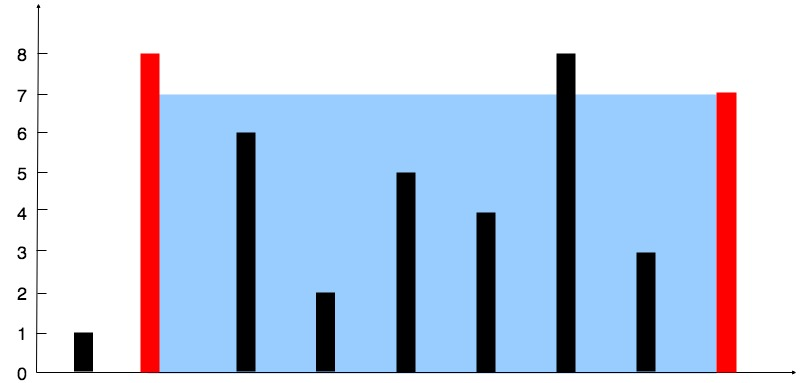## 11. Container With Most Water

Given n non-negative integers a1, a2, ...,an , where each represents a point at coordinate (i, ai).n vertical lines are drawn such that the two endpoints of line i is at (i, ai) and (i, 0). Find two lines, which together with x-axis forms a container, such that the container contains the most water.

Note: You may not slant the container and n is at least 2.The above vertical lines are represented by array [1,8,6,2,5,4,8,3,7]. In this case, the max area of water (blue section) the container can contain is 49.

Example:

``````Input: [1,8,6,2,5,4,8,3,7]

Output: 49
``````

Thoughts:

1. Two pointer: the shorter height need to move since the rest solution is guaranteed less optimal
``````class Solution(object):
def maxArea(self, height):
"""
:type height: List[int]
:rtype: int
"""
left, right = 0, len(height) -1

res = 0
while left <= right:
res = max(res, (right - left) * min(height[left], height[right]))
if height[right] > height[left]:
left += 1
else:
right -= 1

return res
``````
``````class Solution(object):
def maxArea(self, height):
"""
:type height: List[int]
:rtype: int
"""
res, i, j = 0 , 0, len(height) -1

while i < j:
h = min(height[i], height[j])
res = max(res, (j - i)*h)
while i < j and height[i] <= h: i+= 1
while i < j and height[j] <= h: j-= 1

return res
``````